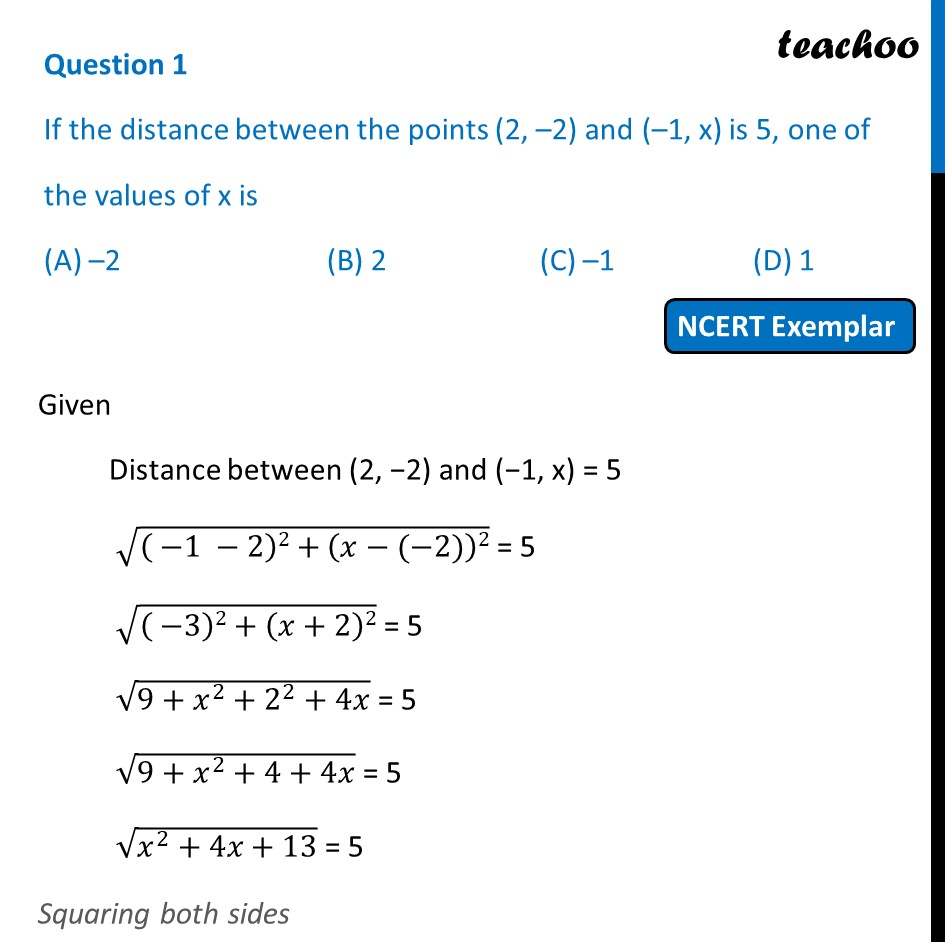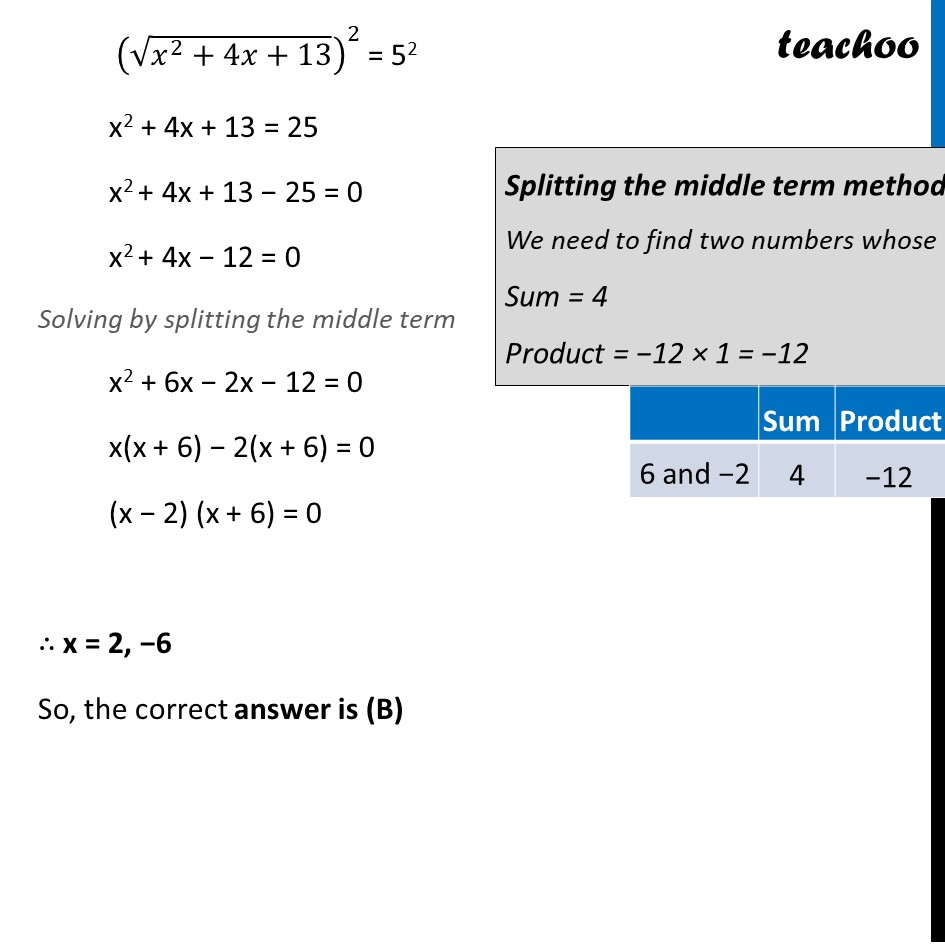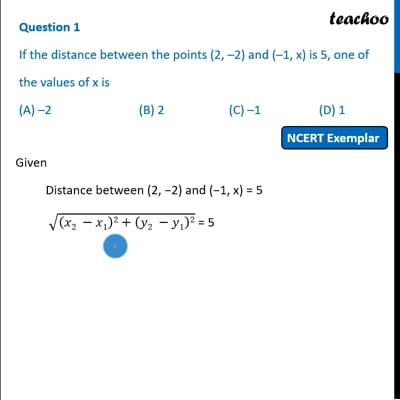NCERT Exemplar - MCQ

Chapter 7 Class 10 Coordinate Geometry
Serial order wise

## (D) 1This video is only available for Teachoo black users

Maths Crash Course - Live lectures + all videos + Real time Doubt solving!

### Transcript

Question 1 If the distance between the points (2, –2) and (–1, x) is 5, one of the values of x is (A) –2 (B) 2 (C) –1 (D) 1 Given Distance between (2, −2) and (−1, x) = 5 √(( −1 −2)2+(𝑥−(−2))2) = 5 √(( −3)2+(𝑥+2)2) = 5 √(9+𝑥^2+2^2+4𝑥) = 5 √(9+𝑥^2+4+4𝑥) = 5 √(𝑥^2+4𝑥+13) = 5 Squaring both sides (√(𝑥^2+4𝑥+13))^2 = 52 x2 + 4x + 13 = 25 x2 + 4x + 13 − 25 = 0 x2 + 4x − 12 = 0 Solving by splitting the middle term x2 + 6x − 2x − 12 = 0 x(x + 6) − 2(x + 6) = 0 (x − 2) (x + 6) = 0 ∴ x = 2, −6 So, the correct answer is (B)